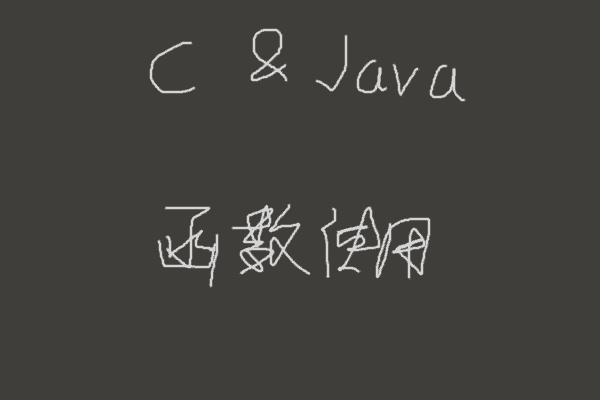# C与JAVA-返回值非空的函数与返回值为空的函数都可单独使用#### 返回值为空的函数可单独使用

printf("%d", printf("%d", printf("这种奇葩玩法也是可以的。")));

static int putSum(int a, int b) {
System.out.println(a+b);
return a+b;
}

int sum = putSum(a, b);

putSum(a, b);

#### 佐证

a = 1;

a = b = 1;

while((pid=fork()) < 0);   // fork()函数创建进程失败会返回-1，若为-1则继续创建直到成功。

int a;
printf("1+14=%d", a=1+14);

int a;
System.out.println("1+14="+(a=1+14));

#### 一点区别

a;
123;